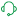Announcements
Product Release Webinar: Qlik Insider airing December 6! REGISTER TODAY!

# Calculating rolling n-period totals, averages or other aggregations

cancel
Showing results for
Did you mean:MVP

## Calculating rolling n-period totals, averages or other aggregations

Last Update:

Nov 30, 2022 6:45:07 AM

Updated By:

Sonja_Bauernfeind

A question that gets asked regularly is how to calculate a rolling measure over a period of N-months (or weeks or days). For example a 12-month rolling total or a 4-week rolling average. There are several ways to do this. But these approaches have some limitations that need to be kept in mind. I'll try to explain these approaches and some of their limitations.

• Accumulation
• RangeXXX functions
• Set analysis
• AsOf tables

First let's load some sample data. The SalesData table below will contain sales amount values for twelve consecutive months.

SalesData:
Month, Amount
1,6
2,4
3,7
4,3
5,4
6,9
7,5
8,7
9,8
10,6
11,9
12,7
];

This is a very simple table with little data, but this enough for demonstration purposes.

Once this data is loaded it's possible to create a straight table chart object to display the amount per month and a running total. As expected Month is used as dimension. The expression sum(Amount) will display the amount per month. Now let's add an expression to calculate a running total over three month periods.

This can be done in two ways. The first uses the Accumulation option for expressions. The same expression sum(Amount) is used, but now the Accumulation option is set to 3 Steps Back: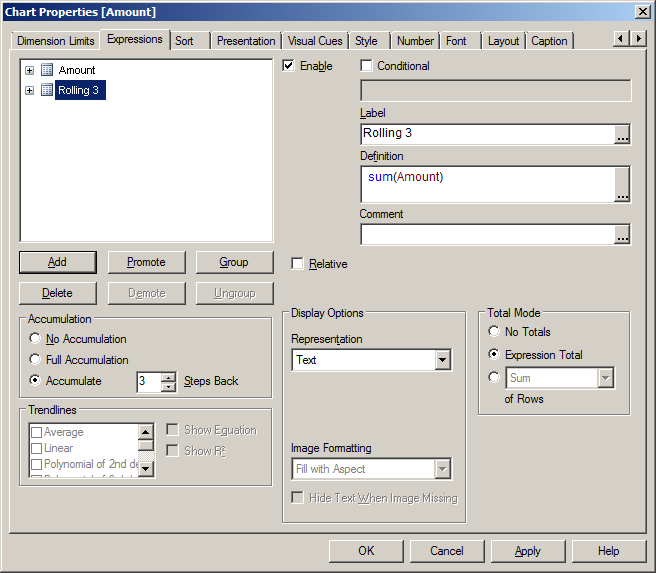The second option uses the rangesum function. That expression looks like this:

rangesum(above(sum(Amount),0,3))

This sums the Amount value on current row and on the previous two rows. The resulting straight table looks like this: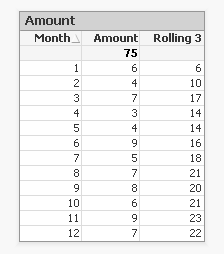This looks good. The rolling 3 months amount is calculated correctly. But what happens if a selection of months is made?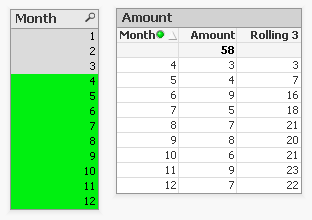The rolling 3 month amount for month 4 is now 3 instead of 14. This is because month 1,2 and 3 are no longer included in the calculation for the rolling 3 month total.

The accumulation option has another issue. It only works when only one dimension is used in the straight table. The rangesum expression can be modified so it can calculate across dimension borders, but the accumulation option can't. The modified rangesum expression adds the total keyword to the above() function:

rangesum(above(total sum(Amount),0,3))

This goes some way to doing what we want, but the issue of displaying the wrong rolling 3 month amount for month 4 isn't solved yet. Contrary to what I first thought there is a solution for this, as Henric pointed out to me in the comments below. By combining the rangesum with the aggr function it's possible to calculate the correct rolling 3 month amounts for each month. The expression needed for that looks like this:

sum(aggr(rangesum(above(total sum({<Month=>}Amount),0,3)),Month))

Read Elif's blog post Accumulative Sums for a more complete explanation.

This expression should calculate the sum of amount for the three month period:

sum({<Month={'>=\$(=only(Month)-2)<=\$(=only(Month))'}>}Amount)

But notice the only() function. This requires that only one month value is selected. After selecting month 4 the result looks like this:This shows the selected month, but also the two previous months. And the values are not accumulated.

sum({<Month={'>=\$(=max(Month)-2)<=\$(=max(Month))'}>}Amount)

That gives a different result, but still not what we're looking for: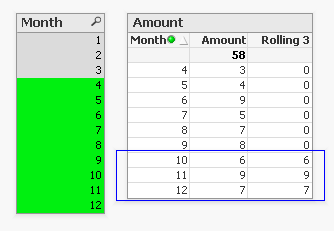Now only the last three months are shown and again the values are not accumulated.

The 'problem' is that the set is calculated once for the entire chart, not per row. This means that it's not possible here to use Month both as a dimension and in the set modifier in the expression.

There's still an option left to discuss: AsOf tables.

The AsOf table links a period with all the periods in the rolling period. In this example months are used, but it can be applied to any type of period like hours, days or weeks.

For the three month periods needed for a rolling 3 month total this means a month should be linked to itself, the previous month and the month before the previous month. The only exceptions are the first month, which is itself the rolling 3 month period, and the second month that together with the first month is its rolling 3 month period. There are no months before the first month so the first two months cannot run over 3 months.

The AsOf table needed for the rolling 3 month calculations looks like this:This table can be created like this:

AsOfMonth:
Month as Month_AsOf,
Month + 1 - IterNo() as Month
Resident SalesData
while IterNo() <= 3;

right join load Month Resident SalesData;

What this does is create three records for every month using the while statement. But that also creates three records for month 1 and 2. This would create a month 0 and a month -1. The right join is used to remove those incorrect month values.

Now that the AsOfMonth table is created the Month_AsOf field can be used instead of the Month field in the straight table. The expression for the straigh table is simply sum(Amount).The straight table now shows the correct rolling 3 month total for month 4.

This can be expanded a little so not only the rolling 3 month can be shown, but also the amount for the month itself. To achieve this the AsOf table is modified by adding a field to label the type of period. And records are added to the table so each Month_AsOf value is linked to the matching Month value:

AsOfMonth:
Month as Month_AsOf,
Month as Month
Resident SalesData;

Concatenate (AsOfMonth)
Month as Month_AsOf,
Month + 1 - IterNo() as Month
Resident SalesData
while IterNo() <= 3;

right join load Month Resident SalesData;

There are now two types of periods available: Current and Rolling 3. Additional period types can be added for example for Rolling 6, Rolling 12 month and Year-to-Date periods. You can find examples of these types in the attached AsOf Table Examples.qvw document.

The period type can be used in the chart expressions to calculate the amount for the wanted period:

Current amount: sum({<Type={'Current'}>}Amount)

Rolling 3 month amount: sum({<Type={'Rolling 3'}>}Amount)

Concluding, there are two solutions that do what we want:

1. The rangesum-aggr combination

2. The AsOf table

The first has the advantage that no changes to the data model are needed. It's also possible to dynamically change the period to aggregate over by using a variable instead of a hardcoded number of periods. A disadvantage is that that it's a somewhat complicated expression that also comes with a performance cost.

The AsOf needs changes in the data model to create the AsOf table and fill it with the necessary records. The advantage is that it likely performs better on large data sets. It's also quite versatile since you can add several sets of records to meet different scenario's. The expressions you end up with in the charts are also less complicated. That said, it will likely take you some time to fully understand the AsOf table concept and realize all the places where you can put it to good use.

In the end you'll have to decide for yourself which solution is appropriate in your situation. With regards to the performance of one or the other solution, you will simply have to test to discover if the performance is acceptable. But of course such testing is already part of your development process, right?

I'd like to thank John Witherspoon for introducing me to the AsOf tables concept and Henric for pointing out the solution using the rangesum function in combination with the aggr function.

Tags (2)
Labels (2)

• ### QlikViewAnonymous
Not applicable

Hi Henric,

is there a way that you could have it showing the true Sum(Amount) for each month instead of it showing the same amount for each month?

ThanksNot applicable

Hi gysbert wassenaar, i'm struggling with this part since the last 1 week

My Requirement is actually this if a user selects the current month the higest 2 values in current month

and i want to see how these higest values are in previous 3 months user can select any month,

Table:

Country, MonthYear, Value

Argentina,Jun2016,550

Japan,Jun2016,200

America,Jun2016,100

America,May2016,300

Argentina,May2016,250

Japan,May2016,150

America,Apr2016,200

Argentina,Apr2016,170

Japan,Apr2016,210

];

My output is below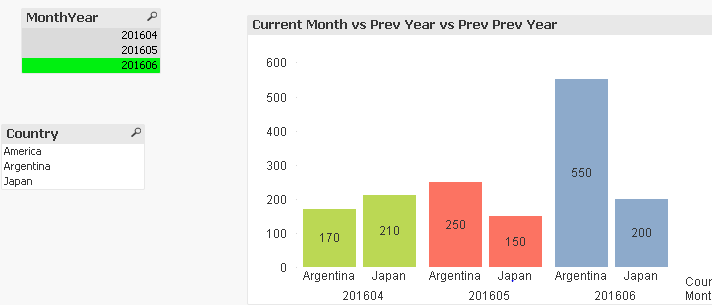Specialist

Hello John,

Avoid posting the same question multiple times.

Top two values in current month comparing the same values with previous month

If required, tag gwassenaar in the same link.Specialist III

Hi,

Am using asoftable to calculate Rolling YTD

PeriodAS as AsOfPeriod
,'YTD'
as PeriodType
,
,
Year(PeriodAS) as Year
RESIDENT PeriodTbl
WHILE iterno() <= num(month(PeriodAS));
;

this gives me from jan to dec but I want t from april to marchCreator III

Hi

I have the rangeavg(above(Count( distinct {\$< Year= >} [OrderID]),0,53))

I want to be able to select either a Financial Year, or Year , without the avg measure above being affected

using e.g Year= within the set analysis does not workContributor III

Excellent post, explanation and examples Gysbert Wassenaar

Gowtham Kesavan, for fiscal year (Apr-Mar) try this:

CONCATENATE (AsOfPeriodTable)

Period as AsOfPeriod

,'FiscalYTD' as PeriodType

,Year(Period) as Year

RESIDENT PeriodTblAnonymous
Not applicable

Hi gwassenaar,

Thanks for this brilliant post!

I am trying to calculate sum of current week plus next three weeks forecast data, but the catch is that each week’s result should be stored to display the value for that week and the line gets created every week in the line chart.

Forecast data gets refreshed weekly and it is archived every week into a NEW QVD (not rewrite the previous QVDs). The reason to archive the data is to store the results of every week to display the Forward Coverage in line chart (shown below). For every week’s QVD I need to sum the forecast data for current week plus the next three weeks.

Formula for Forward Coverage and Line chart:

I need to compare the on-hand inventory data with cumulative 4-week forecast (current week plus next 3 weeks) to calculate the Forward coverage.

Please see the sample data below. I used Technical week as the only dimension, but there are some other product dimensions in the data set.

The on-hand data is CYTD data as shown below.

On_Hand:
Technical_Week, On_Hand_Cumulative
2, -1800
3, 359
4, 20127
5, 115806
7, 304641
8, 496580
9, 557762
]
;

Forecast Data:

Technical_Week, Forecast
9,  68,37
10, 22,790
11, 9,116
12, 505,597
13, 842,661
14, 842,661
15, 505,597

.

.

.

.

.

.

.

57, 2,655

58, 2,655

];

Forward Coverage value for Current Week =

Sum(On_Hand_Cumulative)

/

Sum (this weeks forecast) + sum(next 3 weeks forecast)

I got stuck on how to store the value of forward coverage for each week to create the historical line until today in the line chart.The line gets calculated as the weeks pass through.

Example line chart: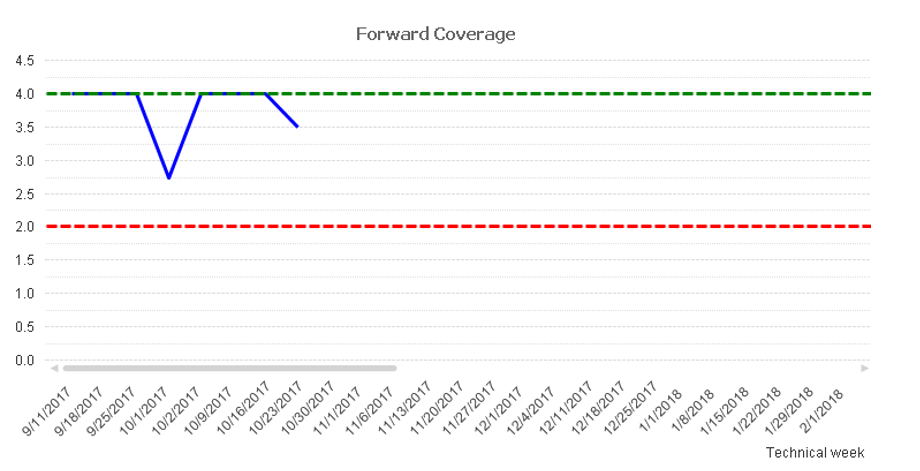Current Technical Week = 9

Current Technical Week Start Date = 10/16/2017

Any assistance is really appreciated. Thanks!Creator III

Hi,

i am trying to use the AsOfMonth table but in your example (the 3 months rolling)

months connected for  04-2017 are

with 03-2017 and 02-2017

what i need is to link the previous 3 months

so for 04_2017 i will link

03-2017, 02-2017, 01-2017

i tried doing it with altering the while loop but couldnt get it right

can anyone thin of how to do it using gwassenaar's example?

thanks

Daniel ChotzenMVP

Daniel, maybe just remove the constant 1 from the second argument to Addmonths() function:

CONCATENATE (AsOfPeriodTable)

//Load Rolling 3 into AsOf table

Period as AsOfPeriod

,'Rolling 3' as PeriodType

,Year(Period) as Year

RESIDENT PeriodTbl

WHILE iterno() <= 3;Creator

Thanks. Very useful post!

Contributors
Version history
Last update:
‎2022-11-30 09:45 AM
Updated by: